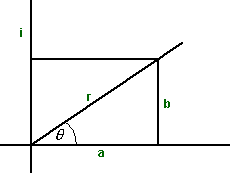# Maths - Complex Arithmetic

Just add the real and imaginary components independently as follows:

(a + i b)+(c + i d) = (a+c) + i (b+d)

## Multiplying complex numbers

To multiply just expand out the terms and group as follows:

(a + i b)*(c + i d) = (a*c - b*d) + i (a*d + b*c)

I don't know if multiplications are so costly in CPU time in modern computers, but if we do want to minimise multiplications we can do a complex multiplication using 3 floating point multiplications as follows:

`multiply(other){double t1= a * other.a;double t2= b * other.b;double t3= (a + b)*(other.a+other.b);a = t1 - t2;b = t3 - t1 - t2;}`

## Norm

This is the distance (r) of a + i b from the origin.

It is written as:

r = | a + i b |

by pythagorous:

r = | a + i b | = math.sqrt(a*a + b*b)

Check that:

|a + i b|*|c + i d| = |a*c - b*d + i (a*d + b*c)|

## Division

We don't tend to use thenotation for division, since complex multiplication is not commutative we need to be able to distinguish between [a][b]-1 and [b]-1[a]. So instead of a divide operation we tend to multiply by the inverse.

In order to calculate the inverse 1/b we multiply top and bottom by its conjugate as follows, conj(b)/b*conj(b). Multiplying a complex number by its conjugate gives a real number and we already know how to divide by a real number.

The conjugate of a + i b is a - i b

so (a + i b)*conj(a + i b) = a*a + b*b

so 1/(a + i b) = a/(a*a + b*b) - i b/(a*a + b*b)

## Representing Rotations using complex numbersinstead of a + i b the complex number could also be represented in what is known as the polar form:

r (cos(θ) + i sin(θ))

in other words replace:

• a = r cos(θ)
• b =r sin(θ)

we can use ei θ = cos(θ) + i sin(θ) to give the exponential form:

r ei θ

If we want combine the result of two rotations, for example rotate by θ1 then rotate by θ2, then we multiply the corresponding complex numbers because:

ei (θ1+θ2) = ei θ1 * ei θ2

Or to combine two rotations by addition if we add the logarithms of the complex numbers.

## Complex Number Calculator

The following calculator allows you to calculate complex arithmetic. Enter the values into the top two complex numbers and then press "+ - or * " to display the result in the bottom number:

 real i
 real i
=
 real i